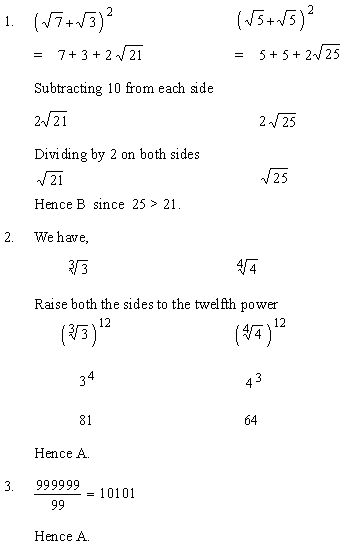Please Support the Monkey! Tell All your Friends and Teachers
 ANSWER EXPLANATIONS Drill : Section IIPerimeter of square is 5 cm ´ 4 lengths = 20 cm Perimeter of the regular pentagon is 4 cm ´ 5 lengths = 20 cm Hence C. To compare speed in each case we also need the distance both from home to school and school to home both of which we cannot assume to be equal. Hence D. PART VI Practice Tests Drill : Section 1 : Verbal Section Section 2 : Quantitative Section Section 3 : Analytical Section Section 4 : Quantitative Section Section 5 : Verbal Section Section 6 : Analytical Section Section 7 : Quantitative Section Answer Explanation To The Drill Section 1 : Verbal Section Section 2 : Quantitative Section Section 3 : Analytical Section Section 4 : Quantitative Section Section 5 : Verbal Section Section 6 : Analytical Section Section 7 : Quantitative Section